## Table of ContentOpen Access

ARTICLE

# A Multi-Attribute Decision-Making Method Using Belief-Based Probabilistic Linguistic Term Sets and Its Application in Emergency Decision-Making

Runze Liu, Liguo Fei*, Jianing Mi

School of Management, Harbin Institute of Technology, Harbin, 150001, China

* Corresponding Author: Liguo Fei. Email:(This article belongs to this Special Issue: Linguistic Approaches for Multiple Criteria Decision Making and Applications)

Computer Modeling in Engineering & Sciences 2023, 136(2), 2039-2067. https://doi.org/10.32604/cmes.2023.024927

## Abstract

Probabilistic linguistic term sets (PLTSs) are an effective tool for expressing subjective human cognition that offer advantages in the field of multi-attribute decision-making (MADM). However, studies have found that PLTSs have lost their ability to accurately capture the views of decision-makers (DMs) in certain circumstances, such as when the DM hesitates between multiple linguistic terms or the decision information is incomplete, thus affecting their role in the decision-making process. Belief function theory is a leading stream of thought in uncertainty processing that is suitable for dealing with the limitations of PLTS. Therefore, the purpose of this study is to extend PLTS to incorporate belief function theory. First, we provide the basic concepts of the extended PLTS (i.e., belief-based PLTS) through case analyses. Second, the aggregation operator of belief-based PLTS is defined with the ordered weighted average (OWA)-based soft likelihood function, which is improved by considering the reliability of the information source. Third, to measure the magnitude of different belief-based PLTSs, the belief interval of singleton is calculated, and the comparison method of belief-based PLTS is constructed based on probabilities. On the basis of the preceding discussion, we further develop an emergency decision framework that includes several novel techniques, such as attribute weight determination and decision information aggregation. Finally, the usefulness of the framework is demonstrated through a case study, and its effectiveness is illustrated through a series of comparisons.

## Graphical Abstract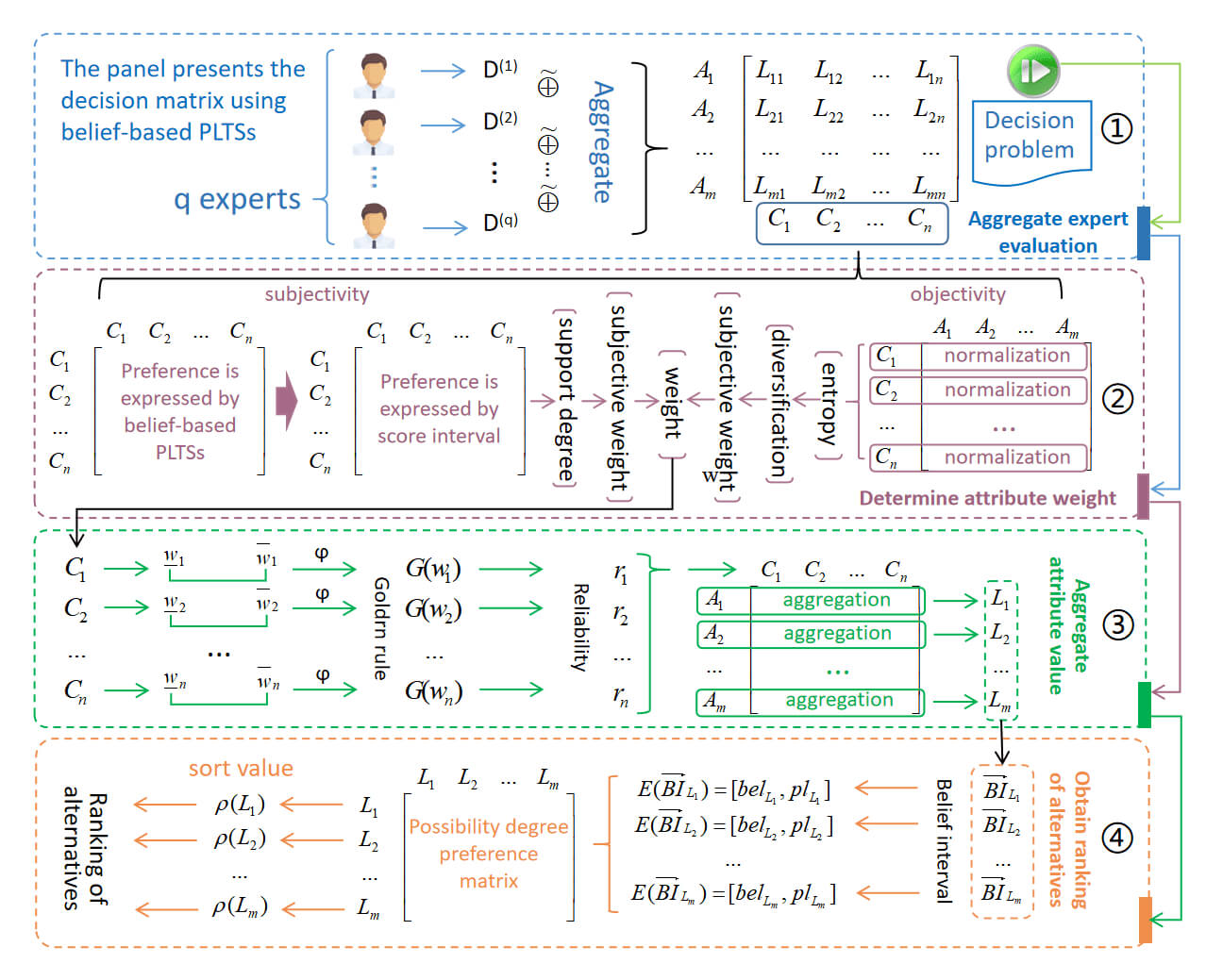## Keywords

1  Introduction

Emergencies of various types are occurring increasingly frequently in the modern interconnected world, which is a phenomenon that has attracted great public attention . Emergencies are characterized by complexity, uncertainty and a high degree of destructiveness. Their occurrence causes great harm in terms of both public health and property damage . Proper awareness of disasters and effective coping strategies can help the public respond such that they do not become life-threatening . Therefore, actively engaging in disaster prevention education is of great significance in minimizing the damage caused by disasters .

Choosing a multiple-disaster risk reduction education plan is an emergency decision problem and therefore essentially a multi-attribute decision problem . Due to the randomness, multi-dimensionality and uncertainty of emergency decision-making, a disaster risk reduction education plan should also consider the complexity of the decision environment . Decision makers are the main participants in emergency decision-making, and their professional knowledge and experience play a key supporting role in evaluating events as they occur. Therefore, human subjectivity will inevitably be a factor in emergency decision-making. To avoid the decision error caused by fuzziness and uncertainty, a wide variety of information expression methods have been proposed to capture DMs’ subjectivity, such as the intuitionistic [7,8], hesitant [9,10], and Pythagorean fuzzy sets , among others.

Because of the lack of accurate information and the environmental uncertainty-as well as the urgency to make a decision-linguistic information and judgment are often employed as they represent the only means through which to represent DMs’ point of view in linguistic terms, such as {bad,medium,good}, which is commonly applied to decision problems . In particular, probabilistic linguistic term sets , which have achieved great success in decision-making, have been proposed as an extension of hesitant fuzzy linguistic term sets as a means to capture information inaccuracy and event randomness.

Since PLTSs were first proposed in , the research on PLTS and MADM based on PLTS has attracted considerable attention. Liao et al.  proposed a comprehensive approach for multi-expert MADM problems that considers both quantitative and qualitative criteria and has been implemented to address green enterprise ranking issues. Jiang et al.  combined PLTS and the fuzzy least absolute regression to construct a fuzzy regression model capable of handling mixed types of inputs. Nie et al.  developed a group decision-making support model using prospect theory-based consistency recovery strategies based on PLTS. Wang et al.  extended TOPSIS, VIKOR and TODIM methods based on PLTS to be applicable to multi-expert MADM issues. An integrated MADM method based on PLTS was presented in  to determine the best medical product supplier. Li et al.  applied PLTS to determine variable weights in solving multi-attribute two-sided matching problems. To evaluate web celebrity shops, Liang et al.  employed long short-term memory and PLTSs to describe customers’ satisfaction. Several novel aggregation operators of PLTSs were defined in  to solve multi-attribute group decision-making problems. A MADM model was presented in  based on incomplete dual probabilistic linguistic preference relations to help enterprises select their 5G partners.

Although traditional PLTSs have been applied to different types of decision problems, the existing approaches show certain limitations and weaknesses, which are reflected in three aspects. The first is in terms of information expression. As probability information is often incomplete, normalization is required in order to fill any gaps in the data [13,23,24]. Second, it only allows for the distribution of probabilities on the singleton and cannot express situations in which the DM hesitates over two or more linguistic terms . Third, the PLTS-based decision methods mostly fail to consider the missing attribute values, which are often unavoidable in practice [12,27,28]. In terms of information aggregation, aggregation operators based on the traditional Dempster’s fusion rule or ER method may suffer from information explosion caused by too many linguistic terms, which implies that the complexity of the algorithm needs to be reduced. In terms of decision-making processes, there is no research on the extension of belief-based PLTSs to emergency management issues, and any related studies do not provide a complete decision-making framework. By recognizing these limitations, the motivation of this study is to overcome these defects to make PLTSs more applicable to long-term decision-making. In particular, as decision environments in many applications, such as responding to major emergencies, are highly uncertain and complex, more powerful tools are needed in order to capture how DMs evaluate information. To achieve such a goal, this paper proposes and develops an uncertain information representation and processing method using belief function theory [29,30], which has been widely used in information fusion  and decision-making .

In short, the purpose of this study is to extend PLTS by incorporating belief function theory to make it more flexible in terms of expressing opinions. The innovations of this paper can be summarized as follows:

•   The new concept of belief-based PLTS is first introduced, and then the composition of its belief interval is proposed, which includes the belief and plausibility functions. Several examples are provided to facilitate understanding of how the extended belief-based PLTS is used.

•   The novel aggregation operator for belief-based PLTS is proposed using an OWA-based soft likelihood function, which can effectively describe DMs subjective attitudes, and the aggregation process is demonstrated using numerical examples. The aggregation operator is upgraded by considering the reliability of information sources, and the aggregation process is demonstrated using numerical examples.

•   To compare different belief-based PLTSs, a novel possibility degree method is defined based on the belief interval of the singleton. An illustrative example is provided to demonstrate how to determine the sizes of different belief-based PLTSs.

•   Based on the proposed belief-based PLTS and its corresponding operations, a multi-attribute decision-making framework is constructed and applied to emergency decision-making, and the detailed process is developed and explained step by step.

To illustrate the applicability of the proposed approach and verify its usefulness, a complete case study is presented based on the defined emergency decision methodology for selecting the best emergency risk education program. Decision results and comparative analysis are given to demonstrate the usefulness and effectiveness of the method proposed in this paper.

The study is carried out according to the following organization. In Section 2, we introduce some preliminaries, including probabilistic linguistic term set, belief function theory, and OWA operator. In Section 3, we explore extending PLTS with belief function theory, introducing basic concepts, aggregation operators, and comparison function. In Section 4, we provide a framework for multi-attribute decision making and apply it to emergency decision problems. In Section 5, we give a case study to demonstrate the usefulness and effectiveness of the proposed emergency decision method. In Section 6, we summarize this study and provide future research.

2  Preliminaries

Some basic prerequisites are introduced in this section, including linguistic term set, probabilistic linguistic term set, belief function theory, and OWA operator.

2.1 Linguistic Term Set (LTS)

In practical decision problems, the linguistic term set is very common and useful, because it is more in line with the DM’s habit of thinking . A subscript-symmetric linguistic term set is defined as S={sα|α=τ,,1,0,1,,τ}, in which τ is a positive integer, and sα satisfies the following characteristics :

•   sα indicates “positive” when α>0, sα indicates “neutral” when α=0, and sα indicates “negative” when α<0;

•   sα>sα^ iff α>α^;

•   the negation operator of sα is defined as neg(sα)=sα.

2.2 Probabilistic Linguistic Term Set (PLTS)

Probabilistic linguistic term set was presented by Pang et al.  to address the weakness that all linguistic term sets in hesitant fuzzy linguistic term set (HFLTS) have the same weight. To demonstrate the shortcomings of HFLTS, and to emphasize the need to introduce PLTS, an illustrative example is presented.

Example 1. In a serious mine accident, the DMs need to evaluate the emergency plans. With regard to an emergency plan ERX, 100 experts use the LTS S={s1=poor,s0=medium,s1=good} to evaluate its feasibility, among which 80 consider it is “good”, and 10 believe it is “medium”, so this case could be expressed as

Feasibility(ERX)={medium(0.1),good(0.8)},(1)

which is not appropriate for HFLTS.

From the above example it can be concluded that the assessment information is expressed not only as a set of possible linguistic terms but also as their corresponding probabilities. To effectively express the opinions of DMs, the probabilistic linguistic term set is proposed and defined as follows:

Definition 2.1.  Let S={sτ,,s1,s0,s1,,sτ} be a LTS, a PLTS is denoted as

L(p)={L(k)(p(k))|L(k)S,p(k)0,k=1,2,,#L(p),k=1#L(p)p(k)1},(2)

where p(k) means the probability of L(k), and #L(p) is the number of nonzero linguistic terms. Note that if k=1#L(p)p(k)1, the normalization process is started. The normalized PLTS is defined as L˙(p)={L(k)(p˙(k))}, where p˙(k)=p(k)/k=1#L(p)p(k).

Based on the above definition, we can represent the evaluation information in Example 1 by a PLTS as L(p)={s0(0.1),s1(0.8)}. The comparison method of two PLTSs was provided in literature .

Definition 2.2.  Let two normalized PLTSs be represented as L1(p) and L2(p), we have

•   If E(L1(p))>E(L2(p)), then L1(p)L2(p);

•   If E(L1(p))=E(L2(p)), then

–  If σ(L1(p))>σ(L2(p)), then L1(p)L2(p);

–  If σ(L1(p))=σ(L2(p)), then L1(p)L2(p),

where E(L(p)) and σ(L(p)) are the score and deviation degree of normalized PLTS L(p) respectively, and their specific definitions are as follows.

Definition 2.3.  Let a normalized PLTS be L(p)={L(k)(p(k)),k=1,2,,#L(p)}, and r(k) be the subscript of L(k), the score of L(p) can be calculated as

E(L(p))=sα¯,(3)

where α¯=k=1#L(p)r(k)p(k).

Definition 2.4.  Let a normalized PLTS be L(p)={L(k)(p(k)),k=1,2,,#L(p)}, r(k) be the subscript of L(k), and E(L(p))=sα¯, then the deviation degree of L(p) can be calculated as

σ(L(p))=k=1#L(p)(p(k)(r(k)α¯))2.(4)

2.3 Belief Function Theory

The theory of belief function is an uncertain reasoning one initiated by Dempster  and inherited and developed by Shafer , therefore also known as Dempster-Shafer theory (DST). After half a century of development, the theory has been well developed and played an important role in a wide variety of fields, such as decision-making  and information fusion . Suppose that the answer to the question of concern consists of a finite set of mutually exclusive elements, called frame of discernement (FoD), expressed as Θ={θ1,θ2,,θn}. The power set of Θ has all of the subsets of Θ, which is expressed as 2Θ={θ1,θ2,,θn,θ1θ2,,Θ}. A piece of evidence or information is defined as a mapping m:2Θ[0,1] that satisfies A2Θm(A)=1 and m(ϕ)=0, also known as the basic belief assignment (BBA). Two significant concepts in the belief function theory are introduced below, namely, belief function and plausibility function.

Definition 2.5. The belief function Bel:2Θ[0,1] of propositions is defined as

Bel(A)=BAm(B),AΘ.(5)

And we have Bel(ϕ)=0 and Bel(Θ)=1.

Definition 2.6. The plausibility function Pl:2Θ[0,1] of propositions is defined as

Pl(A)=1Bel(A¯)=BA=ϕm(B),AΘ,(6)

where A¯=ΘA, and Bel(A)Pl(A). BI(A)=[Bel(A),Pl(A)] forms the belief interval of proposition A [46,47].

2.4 OWA Aggregation Operator

OWA operator, originally introduced by Yager et al. , is an information aggregation method between the maximum and minimum operators.

Definition 2.7.  Let {a1,a2,,an} be a data set. The ordered weighted average operator is defined as

OWA(a1,a2,,an)=j=1nwjbj,(7)

where ω={w1,,wn} is the weighting vector that satisfies wj[0,1] and j=1nwj=1, and bj is the jth largest value in {a1,a2,,an}. Let λ be an index function, and λ(j) is the index of jth largest value, then the OWA operator can be described as

OWA(a1,a2,,an)=j=1nwjaλ(j).(8)

Definition 2.8.  OWA operators are highly dependent on weighting vector ω. When weight distributions are special, several types of OWA operators are expressed as follows.

•   ω:w1=1,wj,j1=0, the OWA operator can be denoted as

OWA(a1,a2,,an)=aλ1=max(ai).(9)

•   ω:wj,jn=1,wn=0, the OWA operator can be denoted as

OWA(a1,a2,,an)=aλn=min(ai).(10)

•   ωn:wj=1/n, the OWA operator can be denoted as

OWA(a1,a2,,an)=1nj=1naj.(11)

•   ωK:wj,jK=0,wK=1, the OWA operator can be denoted as

OWA(a1,a2,,an)=aλK.(12)

Definition 2.9. The weighting vector for OWA operator can be determined as

wj=f(jn)f(j1n),(13)

where f is a monotonic function that satisfies f(0)=0 and f(1)=1.

In , a useful function f(x)=xm, m0 was given, and then a parameter α was proposed to measure the degree of optimism satisfies m=1αα. So for a given α, the weighting vector can be determined as

wj=(jn)1αα(j1n)1αα.(14)

Note that the larger attitudinal character α is, the more optimistic the DM is.

3  Belief-Based Probabilistic Linguistic Term Sets

In this section, the belief-based probabilistic linguistic term sets are developed to overcome the issues of PLTSs by expressing and dealing with more uncertainty. We first give the basic concept of belief-based PLTSs, then provide the aggregation operator and finally define the score function in order to effectively complete the emergency decision-making process.

3.1 The Basic Concept

The probabilistic linguistic term set effectively solves the problem that the HFLTS cannot assign weight to the possible linguistic terms according to the DM’s preference. However, the PLTS has also been criticized for its limitations in decision-making . According to Definition 2.1, PLTS allows probability information to be incomplete, but other operations of the PLTS can only be performed after the probability is normalized. Allowing incomplete information expression is conducive to contain more uncertainty, but the normalization for subsequent calculations directly distributes the uncertainty to each linguistic term, which artificially removes some unknown uncertainties and weakens the effectiveness of the modeling uncertainty.

In complex decision-making, especially emergency problems, there may be various uncertainties, including randomness, vagueness and incompleteness . Randomness comes from non-deterministic conditions, ambiguity is caused by unclear classification of things, and incompleteness is caused by incomplete information. PLTS has some potential limitations in the expression of uncertainty, such as the aforementioned normalization problem, the inability to express simultaneity, and the lack of attribute values that are not considered. The belief function theory is a powerful tool for expressing and dealing with uncertainty, which has been widely declared and recognized by authoritative literature [29,34,35,46]. In order to solve the potential limitation of PLTS in MADM, the concept of belief-based PLTS is introduced.

For instance, in Example 1, the evaluation is represented by PLTS as Feasibility(ERX)={medium(0.1),good(0.8)}, in which 10 experts have not expressed their opinions. This part of information can be regarded as unknown or uncertain. Based on belief function theory, the evaluation can be represented as

Feasibility1(ERX)={medium(0.1),good(0.8),{poor,medium,good}(0.1)}={s0(0.1),s1(0.8),S(0.1)},(15)

where S is FoD, indicating unknown or uncertain.

It is worth noting that incompleteness of information is also seen in another scenario in decision making. For example, in multi-attribute emergency decision making, the DM has completed the evaluation of the performance of an emergency plan under other attributes, but only in the aspect of attribute “response speed” is unknown. The traditional PLTS-based emergency decision making method rarely considers this situation, which leads to the interruption of the emergency procedure. Within the frame of belief function theory, the missing attribute value can be represented as S(1). The ability of the belief function theory to express uncertain information can ensure that the decision can proceed even with the incomplete information.

Another motivation that we have to extend PLTS with belief function theory is to deal with a decision scenario where a DM is hesitant between two or more linguistic terms. In other words, the probability distribution on the single term no longer meets the expression requirements of DMs, but needs to allocate the belief on multiple subsets. Take the decision scenario in Example 1, where 80 experts evaluate it “good”, 10 evaluate it “medium”, and 10 hesitate between the terms “good” and “medium”. Obviously, PLTS is powerless to describe this case, but the theory of belief function can handle and represent such a case well with the expression

Feasibility2(ERX)={medium(0.1),good(0.8),{medium,good}(0.1)}={s0(0,1),s1(0.8),s0s1(0.1)}.(16)

Through the above analysis and discussion, we identify some limitations of PLTS in expressing DMs’ views, and analyze the advantages of belief function theory in solving these problems. This is therefore an appropriate time to present the belief-based probabilistic linguistic term set as below:

Definition 3.1. Let S={sτ,,s1,s0,s1,,sτ} be a LTS, a belief-based PLTS is defined as

={β(ι)|ι2S,β(ι)0,ι2Sβ(ι)=1},(17)

where β(ι) means the basic belief assignment (BBA) of proposition ι (a subset of LTS S). Note that the belief-based PLTS is denoted by β for convenience.

Based on Definition 3.1, the evaluations in Eqs. (15) and (16) can be represented by belief-based PLTSs as 1={s0(0.1),s1(0.8),S(0.1)} and 2={s0(0.1),s1(0.8),s0s1(0.1)}, which can also be written as β1(s0)=0.1,β1(s1)=0.8,β1(S)=0.1 and β2(s0)=0.1,β2(s1)=0.8,β2({s0,s1})=0.1. According to the above definitions and examples, we can conclude that the belief-based PLTS has the following desired properties and advantages: (1) for PLTS with incomplete information, the operation of normalization is no longer necessary, and the uncertainty is represented by the universal set (i.e., LTS S, can also be interpreted as FoD), thus unifying the information expression mode based on linguistic term sets from the mathematical form (2) belief-based PLTS has the ability to capture DMs’ hesitations among possible linguistic terms, can more fully allow DMs to express opinions. It is worth noting that belief-based PLTS degrades to the traditional PLTS when the belief is all assigned to the singleton, i.e., it is backward compatible. Naturally, the belief function and plausibility function of belief-based PLTS can be defined.

Definition 3.2. The belief function of belief-based PLTS Bel:2S[0,1] is defined as

Bel(A)=BAβ(B),AS.(18)

And we have Bel(ϕ)=0 and Bel(S)=1.

Definition 3.3. The plausibility function of belief-based PLTS Pl:2S[0,1] is defined as

Pl(A)=1Bel(A¯)=BA=ϕβ(B),AS,(19)

where A¯=ΘA, and Bel(A)Pl(A). So BI(A)=[Bel(A),Pl(A)] forms the belief interval of proposition A.

3.2 A Novel Aggregation Operator for Belief-Based PLTS

In decision problems, an aggregation operator is inevitably employed, which is an approach combining two or more single information expressions. The above manifests the advantages of the belief-based PLTS in representing uncertain information. To make it exert maximum in decision making, this section provides a novel aggregation operator for belief-based PLTS ground on soft likelihood function.

Soft likelihood function was originally proposed by Yager et al.  for calculating likelihood functions of probabilistic evidence in the context of forensic crime investigations. It breaks the limitations of traditional likelihood functions that use logical “anding” to aggregate elements, and has been extended to uncertain decision environments by D numbers , power OWA [55,56], and Pythagorean fuzzy sets [57,58]. With the flexible and reliable combination ability of soft likelihood function, in this section, an aggregation operator for belief-based PLTS is proposed.

Definition 3.4. Let ={1,2,,n} be n belief-based PLTSs on S={sτ,,s1,s0,s1,,sτ}. The likelihood value of ιiS is defined as

Γιi=j=1nβj(ιi).(20)

The above definition multiplies the belief of ι in each belief-based PLTS to determine the value of aggregated ι. It is easy to find that this definition is a logical “and”, that is, the aggregated ι depends on the minimum ι in each belief-based PLTS. This means, however, that no matter how many belief-based PLTSs have a large ι value, Γιi=0 as long as βj(ιi)=0 in one belief-based PLTS, which is obviously not true in most applications. This kind of likelihood function is called hard likelihood function. To fix the defect, a soft likelihood function based on OWA operator has been developed , which is used to define the aggregation operator for belief-based PLTS.

Definition 3.5. Let λιi be an index function of ιiS, and λιi(k) indicate the index of the kth largest belief of ιi in . The product operation of the j largest belief of ιi can be defined as

Prodιi(j)=k=1jβλιi(k)(ιi),(21)

where βλιi(k)(ιi) is the kth largest belief of ιi. Note that when j=n, Eq. (21) degenerates into the hard likelihood function, i.e., Prodιi(n)=Γιi. In addition, it is easy to tell that Prod is a monotonically decreasing function, i.e., if j1j2, then Prod(j1)Prod(j2).

Definition 3.6. The OWA-based soft likelihood function of ιiS is defined as

Γιi,OWA=OWA(Prodιi(1),Prodιi(2),,Prodιi(n))=j=1nwjProdιi(j),(22)

where wj[0,1] is the weighting vector that satisfies j=1nwj=1.

According to Definition 2.8, we analyze the OWA-based soft likelihood function corresponding to the special forms of weight distribution.

•   For ω:w1=1,wj,j1=0, Γιi,OWA=Prodιi(1)=βλιi(1)(ιi). In this case, the aggregated belief of ιi is equal to the largest one in .

•   For ω:wj,jn=1,wn=0, Γιi,OWA=Prodιi(n)=j=1nβj(ιi), which degenerates into the hard likelihood function.

•   For ωn:wj=1/n, Γιi,OWA=1nj=1nProdιi(j)=1nj=1n(k=1jβλιi(k)(ιi)), which is a simple average of Prodιi(j).

Definition 3.7. Based on Definition 2.9, the attitudinal character α can be employed to calculate the soft likelihood value of ιiS. Therefore the aggregated belief of ιi can be calculated as

Γιi,α=j=1n((jn)1αα(j1n)1αα)k=1jβλιi(k)(ιi),ιiS.(23)

To ensure that the aggregated result is still a belief-based PLTS, it needs to be normalized as

ιi(βα)=Γιi,αιiSΓιi,α.(24)

Therefore, the aggregation operator of n belief-based PLTSs can be defined as

12n={ιi(βα)|ιi2S,βα(ιi)0,ιi2Sβα(ιi)=1}.(25)

To demonstrate the usage of the above-proposed aggregation operator, the following example is given.

Example 2. Let S={s1,s0,s1} be a LTS, and five belief-based PLTSs on S be represented by Definition 3.1 as 1={s1(0.7),s0(0.2),s0s1(0.1)}, 2={s1(0.55),s0(0.25),s1(0.2)}, 3={s1(0.8),s0s1(0.2)}, 4={s1(0.5),s0(0.2),s0s1(0.3)}, and 5={s1(0.5),s0(0.2),s0s1(0.3)}. We take s1 as an example to show the solution of OWA-based soft likelihood function.

According to Definition 3.5, the product of the j largest belief of s1 can be calculated as Prods1(1)=βλs1(1)(s1)=β3(s1)=0.8, Prods1(2)=βλs1(1)(s1)×βλs1(2)(s1)=β3(s1)×β1(s1)=0.8×0.7=0.56, Prods1(3)=βλs1(1)(s1)×βλs1(2)(s1)×βλs1(3)(s1)=β3(s1)×β1(s1)×β2(s1)=0.8×0.7×0.55=0.3080, Prods1(4)=βλs1(1)(s1)×βλs1(2)(s1)×βλs1(3)(s1)×βλs1(4)(s1)=β3(s1)×β1(s1)×β2(s1)×β4(s1)=0.8×0.7×0.55×0.5=0.1540, Prods1(5)=βλs1(1)(s1)×βλs1(2)(s1)×βλs1(3)(s1)×βλs1(4)(s1)×βλs1(5)(s1)=β3(s1)×β1(s1)×β2(s1)×β4(s1)×β5(s1)=0.8×0.7×0.55×0.5×0.5=0.0770.

Now we discuss the soft likelihood value of s1 with different α.

•   When α=0.1, based on Eq. (14), the weighting vector is ω=(0,0.0003,0.0098,0.1241,0.8658)T, the soft likelihood value can be obtained by using Eq. (23) as Γs1,0.1=0×0.8+0.0003×0.56+0.0098×0.3080+0.1241×0.1540+0.8658×0.0770=0.0890.

•   When α=0.5, based on Eq. (14), the weighting vector is ω=(0.2,0.2,0.2,0.2,0.2)T, the soft likelihood value can be obtained by using Eq. (23) as Γs1,0.5=0.2×0.8+0.2×0.56+0.2×0.3080+0.2×0.1540+0.2×0.0770=0.3798.

•   When α=0.9, based on Eq. (14), the weighting vector is ω=(0.8363,0.0670,0.0416,0.0306,0.0245)T, the soft likelihood value can be obtained by using Eq. (23) as Γs1,0.9=0.8363×0.8+0.0670×0.56+0.0416×0.3080+0.0306×0.1540+0.0245×0.0770=0.7260.

According to the above process, soft likelihood values of other propositions (s0, s1, and {s0s1}) under different α can be calculated and normalized by using Eq. (31). The results are manifested in Table 1. The conclusion is that (1) when α=0.1, 15={s1(0.9911),s0(0.0045),s0s1(0.0045)}, (2) when α=0.5, 15={s1(0.6732),s0(0.1106),s1(0.0709),s0s1(0.1453)}, (3) when α=0.9, 15={s1(0.5500),s0(0.1613),s1(0.1268),s0s1(0.1619)}.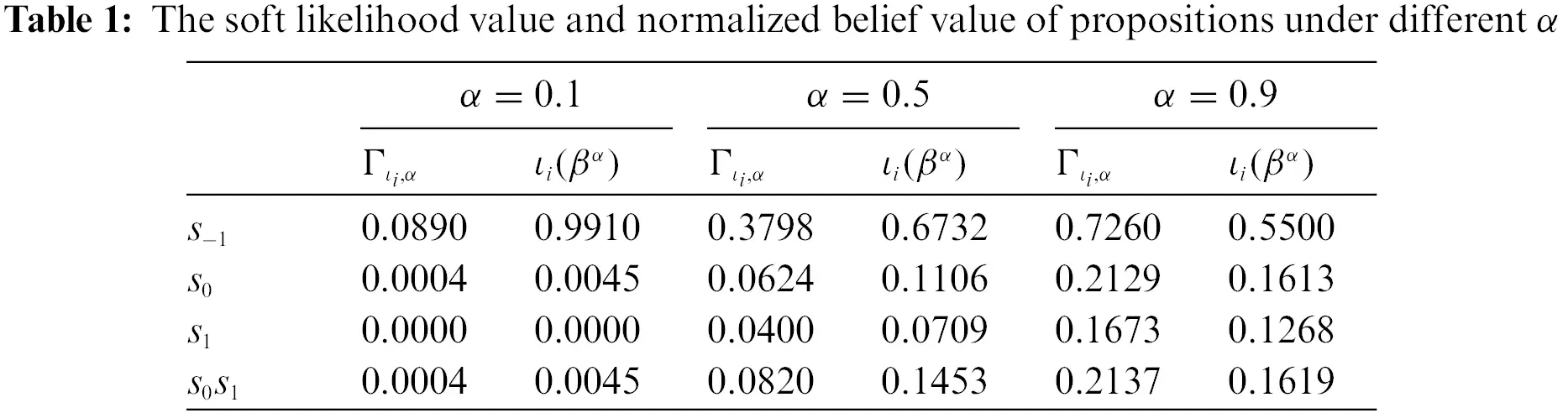3.3 The Aggregation Operator Considering the Reliability of Belief-Based PLTS

In practical decision problems, different belief-based PLTSs generally have different importance, i.e., weight, also known as reliability. The aggregation operator proposed in the previous section assumes that all belief-based PLTSs have the same reliability, which leads to certain limitations in decision making. To solve this problem, this section develops an aggregation operator considering reliability.

From the definitions in the previous section, it can be concluded that the advantages of soft likelihood function-based aggregation operator mainly come from two aspects: cumulative multiplication and OWA. Reliability will affect the order determination and weight vector generation.

Definition 3.8. The reliability of {1,2,,n} is denoted as {r1,r2,,rn} that satisfies rj[0,1] and j=1nrj=1. Let γιi be an index function of β(ιi)×r, and γιi(k) indicate the index of the kth largest product of β(ιi)×r. The product operation of the j largest belief of ιiS is defined as

Prod~ιi(j)=k=1jβγιi(k)(ιi),(26)

where βγιi(k)(ιi) is the kth largest belief of β(ιi)×r. Note that Prod~ is also a monotonically decreasing function, i.e., if j1j2, then Prod~(j1)Prod~(j2).

Definition 3.9. The OWA-based soft likelihood function of ιiS considering reliability is defined as

Γ~ιi,OWA=OWA(Prod~ιi(1),Prod~ιi(2),,Prod~ιi(n))=j=1nw~jProd~ιi(j),(27)

where w~j[0,1] and j=1nw~j=1 is the weighting vector.

Definition 3.10. To determine the OWA weighting vector, the sum of reliability of the j largest β(ιi)×r product is defined as

χj=k=1jrγιi(k).(28)

Consequently, the OWA weighting vector of the soft likelihood function considering reliability can be defined as

w~j=(χj)1αα(χj1)1αα.(29)

Note that if the reliability of all belief-based PLTSs is equal, i.e., rj=1/n, ω~ is reduced to ω.

Definition 3.11. The aggregation operator of n belief-based PLTSs considering reliability is defined as

1~2~~n={ιi(βα)|ιi2S,βα(ιi)0,ιi2Sβα(ιi)=1},(30)

where ιi(βα) is defined as

ιi(βα)=Γ~ιi,αιiSΓ~ιi,α.(31)

The case in Example 2 is used to demonstrate the aggregation operator considering reliability.

Example 3. Let the reliability of {1,,5} be {r1=0.35,r2=0.25,r3=0.15,r4=0.2,r5=0.05}. Let’s take s1 as an example, because β1(s1)×r1=0.1050, β2(s1)×r2=0.1375, β3(s1)×r3=0.2800, β4(s1)×r4=0.1000, and β5(s1)×r5=0.0250, so γs1(1)=3, γs1(2)=2, γs1(3)=1, γs1(4)=4, and γs1(5)=5. Based on Definition 3.8, the product of the j largest belief of s1 can be calculated as Prod~s1(1)=β3(s1)=0.8, Prod~s1(2)=β3(s1)×β2(s1)=0.44, Prod~s1(3)=Prod~s1(2)×β1(s1)=0.3080, Prod~s1(4)=Prod~s1(3)×β4(s1)=0.1540, and Prod~s1(5)=Prod~s1(4)×β5(s1)=0.0770. According to Definitions 3.9 and 3.10, the soft likelihood values of s1 with different α are calculated considering reliability, and the results are manifested in Table 2.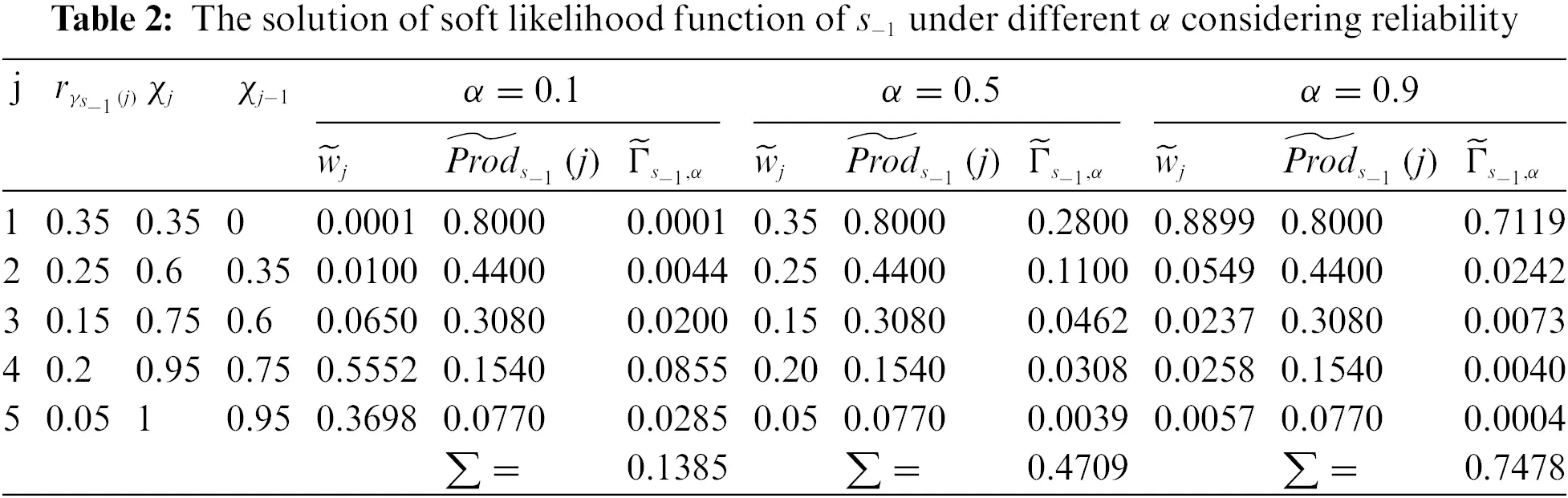According to the above process, soft likelihood values of other propositions (s0, s1, and {s0s1}) under different α can be calculated and normalized by using Definition 3.11. Finally, the conclusion is that (1) when α=0.1, 1~~5={s1(0.9802),s0(0.0134),s1(0.0036),s0s1(0.0028)} (2) when α=0.5, 1~~5={s1(0.6661),s0(0.1197),s1(0.1132),s0s1(0.1010)} (3) when α=0.9, 1~~5={s1(0.5335),s0(0.1291),s1(0.1560),s0s1(0.1814)}.

In Examples 2 and 3, we present several special cases to reflect the impact of parameter α on the result of aggregation. In order to further highlight the effect, the experiment is carried of aggregation operator based on soft likelihood function (with ans without) considering reliability. As can be seen from Fig. 1, with the increase of α, the belief of s1 is decreasing while the other belief is increasing, from which we can see the influence of DM’s attitude on the aggregation result.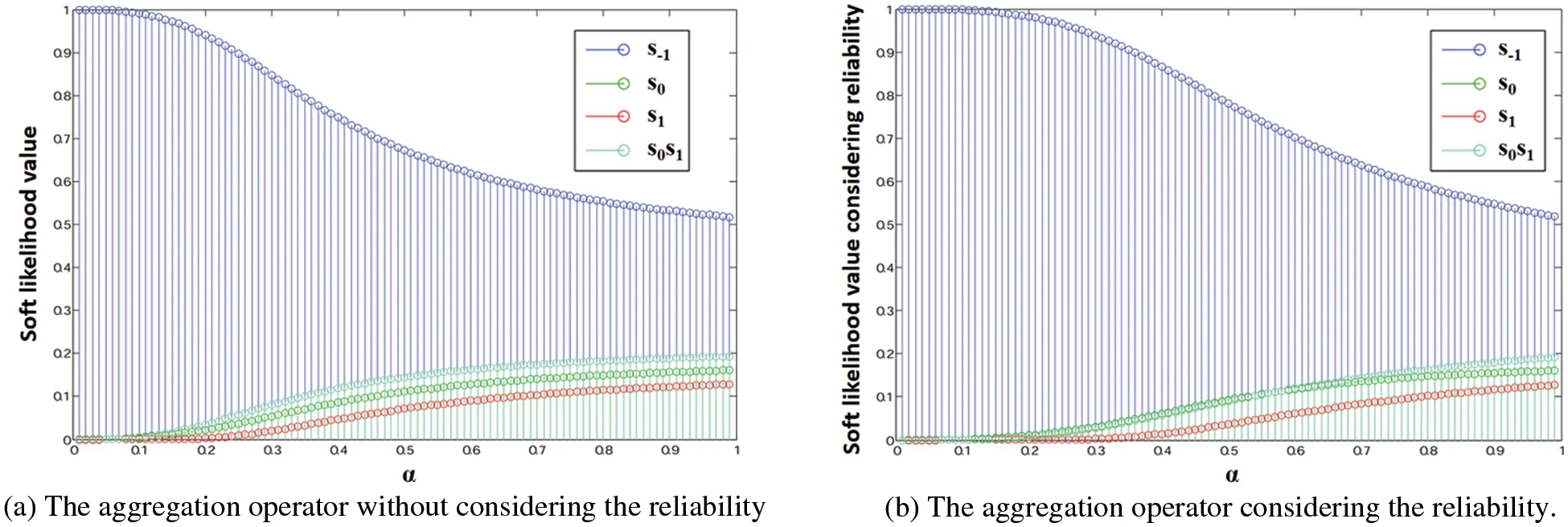Figure 1: The belief distribution of the aggregated belief-based PLTS with different α

3.4 The Comparison between Belief-Based PLTSs

Belief-based PLTS is a new expression of uncertainty based on linguistic information. Due to its complexity, it is not easy to define score functions like PLTS. Therefore, by virtue of belief interval, we propose a novel possibility degree method for comparing belief-based PLTSs.

Definition 3.12. Let be a belief-based PLTS on S={sτ,,s1,s0,s1,,sτ}, the belief interval of each singleton constitutes a belief interval vector of , expressed as

BI=(BI(sτ),,BI(s1),BI(s0),BI(s1),,BI(sτ))T,(32)

where BI(sα)=[Bel(sα),Pl(sα)] means the belief interval of linguistic term sα.

Definition 3.13. Let BI be the belief interval vector of belief-based PLTS , the score of BI can be calculated as

E(BI)=[αSαBel(sα),αSαPl(sα)]=[bel,pl].(33)

Definition 3.14. Let 1 and 2 be two belief-based PLTSs, BI1 and BI2 be their belief interval vector, and the corresponding scores be E(BI1)=[bel1,pl1] and E(BI2)=[bel2,pl2], the possibility degree for 12 can be defined as

p(12)=min{I1+I2,max{pl1bel2,0}}I1+I2,(34)

where I1=pl1bel1 and I2=pl2bel2. The order relationship between 1 and 2 is denoted by 12p.

Based on above definitions, under the assumption that 1, 2, 3 are three belief-based PLTSs and their scores are E(BI1)=[bel1,pl1], E(BI2)=[bel2,pl2], E(BI3)=[bel3,pl3], four theorems as below can be easily deduced.

Theorem 3.1. p(12)[0,1].

Theorem 3.2. p(12)=1 iff pl2bel1.

Theorem 3.3. p(12)=0 iff pl1bel2.

Theorem 3.4. p(12)+p(1<2)=1. Particularly, p(11)=0.5.

The above theorems are obvious, so the proof is omitted. Definition 3.14 provides the possible degree calculation method for two belief-based PLTSs sorting, and we further extend it to the scenario of n belief-based PLTSs.

Definition 3.15. Let a set of belief-based PLTSs with n elements be represented as ={1,2,,n}, and the score of i is E(BIi)=[beli,pli], i{1,,n}. The possible degree matrix of can be obtained as P=(pij)n×n, where pij=p(ij), i,j. Matrix P contains the possible degree relations among all belief-based PLTSs, so sorting is transformed to solve the sorting vector problem of matrix P. Based on , the sort value of belief-based PLTS i can be calculated as

ρ(i)=1n(n1)(j=1npij+n21),i,(35)

which can be employed to sort .

The belief-based PLTSs in Example 2 are used to be sorted by using the above method in this section.

Example 4. We take 1 as an example to demonstrate the calculation of its score of belief interval vector. Based on Definitions 3.2, 3.3 and 3.12, the belief interval vector of 1 can be calculated as

BIL1=(BIL1(s1),BIL1(s0),BIL1(s1))T=([BelL1(s1),PlL1(s1)],[BelL1(s0),PlL1(s0)],[BelL1(s1),PlL1(s1)])T=([0.7,0.7],[0.2,0.3],[0,0.1])T.(36)

Then the score of BI1 can be calculated by using Definition 3.13 as

E(BI1)=[bel1,pl1]=[(1)0.7+00.2+10,(1)0.7+00.3+10.1]=[0.7,0.6].(37)

So the scores of 1,,5 can be obtained as E(BI2)=[0.35,0.35], E(BI3)=[0.8,0.6], E(BI4)=[0.5,0.2], and E(BI5)=[0.5,0.2], respectively. The possible degree between two belief-based PLTSs is calculated based on Definition 3.14 below. Taking 1 and 2 as an example, p(12)=0 can be obtained. Then the possible degree matrix can be obtained as

P=(pij)5×5[1/202/30011/211/21/21/301/20011/211/21/211/211/21/2].(38)

According to Definition 3.15, the sort value of the belief-based PLTSs can be calculated as ρ(1)=0.1333, ρ(2)=0.25, ρ(3)=0.1167, ρ(4)=0.25, and ρ(5)=0.25. So the order can be determined as 20.540.55112/33.

4  Emergency Decision-Making Based on Belief-Based Probabilistic Linguistic Term Sets

In recent years, various unconventional emergencies have occurred frequently, which has become a major risk and disaster facing mankind. How to reduce the loss caused by a disaster and carry out effective rescue work based on emergency decision methods has become an important step in dealing with emergencies. In this section, we propose a multi-attribute decision making method for emergency decision based on the defined belief-based PLTS.

4.1 Problem Description

In a multi-attribute emergency decision making problem, the alternative set is {A1,A2,,Am}, the attribute is represented as {C1,C2,,Cn}, the corresponding weight is {w1,w2,,wn}, the set of decision experts is {E1,E2,,Eq}, with weight {g1,g2,,gq}. Experts use belief-based PLTSs to evaluate alternatives under different attributes, and the resulting decision matrix is expressed as

D(k)=(ijk)m×n=[11k12k1nk21k22k2nkm1km2kmnk]=[β11kβ12kβ1nkβ21kβ22kβ2nkβm1kβm2kβmnk],(39)

where ijk indicates the evaluation of alternative Ai under attribute Cj from expert Ek.

4.2 Aggregation of Decision Information of Experts

After obtaining the experts’ decision matrices, the opinions need to be aggregated first. For the comprehensive performance of alternative Ai under attribute Cj, the evaluations provided by experts are aggregated by using the aggregation operator considering reliability proposed in Subsection 3.3. Note that the reliability here is the weight of the expert. According to Definition 3.11, the aggregated evaluation is calculated as ij=ij1~ij2~~ijq, so the aggregated decision matrix is expressed as

D=(ij)m×n[11121n21222nm1m2mn],(40)

where ij denotes the aggregated belief-based PLTS of ij1,,ijq.

4.3 Determination of Attribute Weight

The methods for determining attribute weights can be divided into the subjective, objective and combination weighting methods. The last method combines the characteristics of the former two, avoids the subjective arbitrariness, and captures the preference of DMs for the attribute. Therefore, based on the defined belief-based PLTS, this paper proposes a combination weighting method which aggregates both subjective and objective.

4.3.1 Determination of Subjective Weight Based on Belief-Based PLTSs

Humans are at a loss when asked to compare multiple attributes. But it is much easier for humans to compare pairs of attributes, an idea that has been repeated in the AHP and BWM approaches . Based on this consideration, we propose a method to determine the subjective weight of attributes.

Let the set of attributes be represented as {C1,C2,,Cn}. The pairwise comparison between Ci and Cj is expressed as a belief-based PLTS ij on S={sτ,,s1,s0,s1,,sτ}. ij is the degree to which attribute Ci is better than attribute Cj, and if α>0 (subscript of sα) indicates that Ci is superior to Cj, and vice versa. In particular, α=0 indicates that Ci is equally important to Cj, so the belief-based PLTS can be obtained as ii𝒞={s0(1)}. Referring to literature [36,61], the concept of the negation of belief-based PLTSs is defined to express the comparison between Cj and Ci based on ij𝒞.

Definition 4.1. For a given belief-based PLTS 𝒞 on S, its negation ¯𝒞 is defined as

β¯(ι)={β(sα),ι=Ssαs,|s|1β(s),ι=S(41)

where sα is a singleton, and s is the focal element which has a cardinality greater than 1.

To demonstrate how to use the defined negation of belief-based PLTSs, the following example is given.

Example 5. The pairwise comparison between Ci and Cj is expressed by a belief-based PLTS ij𝒞 on S={s1,s0,s1}, then the comparison between Cj and Ci can be calculated in the following cases based on the above definition.

•   Case 1: when ij𝒞={s1(1/3),s0(1/3),s1(1/3)}, based on Eq. (41), we have ji𝒞={s0s1(1/3),s1s1(1/3),s1s0(1/3)}.

•   Case 2: when ij𝒞={s1(0.7),s0s1(0.3)}, based on Eq. (41), we have ji𝒞={s1s0(0.7),S(0.3)}.

•   Case 3: when ij𝒞={s0(1)}, which is a special case, indicating that Ci is of equal importance to Cj. By using Eq. (41), we have ji𝒞={s1s1(1)}, which means that belief-based PLTSs {s0(1)} and {s1s1(1)} have the same semantics in this case.

A pairwise comparison matrix 𝒞=(ij𝒞)n×n can be obtained based on the above definitions, and the actual number of comparisons of the DM is n(n1)2. Then the score of belief interval vector of ij𝒞 can be calculated by using Definition 3.13 as [belij𝒞,plij𝒞]. To make all the values participating in the normalization positive, the scores will be shifted to ensure that the minimum lower limit of the interval values corresponding to each attribute is 0, which is expressed as [bel~ij𝒞,pl~ij𝒞]. Then the support degree of attribute Ci can be determined as [supibel~,supipl~]=[j=1,jinbelij𝒞,j=1,jinplij𝒞]. Therefore, the subjective weight of attribute Ci is denoted by an interval value as [w_is,w¯is]=[supibel~/i=1nsupipl~,supipl~/i=1nsupipl~].

4.3.2 Determination of Objective Weight Based on Interval Entropy Weight Method

The basic idea of the entropy weight method is to determine the objective weight according to the magnitude of the attribute variability. Generally, entropy is employed to reflect uncertainty. In decision-making problems, the lower the entropy, the greater the uncertainty, the greater the degree of variation of the attribute, the greater the degree of differentiation, and the greater the weight, and vice versa.

For decision matrix D=(ij)m×n shown in Eq. (40), the score of belief interval vector of ij can be calculated by using Definition 3.13 as

(Eij)m×n=(E(BIij))m×n=[[bel11,pl11][bel12,pl12][bel1n,pl1n][bel21,pl21][bel22,pl22][bel2n,pl2n][belm1,plm1][belm2,plm2][belmn,plmn]].(42)

To make all the values participating in the normalization positive, the scores will be shifted to ensure that the minimum lower limit of the interval values corresponding to each attribute is 0. The interval entropy weight method is carried out in the following steps.

Step 1: Normalize data. Since there may be negative numbers in the matrix, we first translate the data to get (Eˇij)m×n, and ensure that the minimum interval number under each attribute is 0. For each attribute, we compute the interval E~ij=[bel~ij,pl~ij] by linear normalization as

bel~ij=belˇiji=1mbelˇij,pl~ij=plˇiji=1mplˇij.(43)

Step 2: Calculate the entropy of E~ij based on Shannon entropy as

SEjbelˇ=min{1ln(m)i=1mbelˇijln(belˇij),1ln(m)i=1mplˇijln(plˇij)},SEjplˇ=max{1ln(m)i=1mbelˇijln(belˇij),1ln(m)i=1mplˇijln(plˇij)}.(44)

Step 3: Calculate the diversification of [SEjbelˇ,SEjplˇ] as

divjbelˇ=1SEjplˇ,divjplˇ=1SEjbelˇ.(45)

Step 4: Obtain the objective weight [w_jo,w¯jo] as

w_jo=divjbelˇjndivjplˇ,w¯jo=divjplˇjndivjplˇ.(46)

4.3.3 Determination of Final Weight by Combining Subjectivity and Objectivity

The subjective weight wjs=[w_js,w¯js] and objective weight wjo=[w_jo,w¯jo] of attribute Cj are obtained in Sections 4.3.1 and 4.3.2, then the comprehensive weight wj=[w_j,w¯j] of attribute Cj can be calculated by combining subjectivity and objectivity as

w_j=w_jsw_joj=1nw¯jsw¯jo,w_j=w¯jsw¯joj=1nw¯jsw¯jo.(47)

4.4 Decision Information Aggregation

Based on the attribute weights obtained in the previous subsection and the decision matrix in Eq. (40), the evaluations of alternative Ai under different attributes needs to be aggregated based on the aggregation operator provided in Section 3.2. Since the attribute weight obtained is given in the form of intervals, we first propose a transformation method to map them to real values. The golden rule is an appropriate alternative for mapping interval numbers to real numbers, which has been used and developed in many literature . The interval weight conversion method based on the golden rule is defined below.

Definition 4.2. Let the interval weight of attribute Cj be wj=[w_j,w¯j], the representation of wj can be defined based on golden rule as

𝒢(wj)=m(wj)+φr(wj)2,(48)

where m(wj)=(w_j+w¯j)/2 is the midpoint of the interval wj, and r(wj)=w¯jw_j is the range of wj. φ[1,1] denotes the attitude of DMs, φ>0 means that the DM is positive, the closer it is to 1, the more positive the DM is, and vice versa, especially φ=0 indicates that the DM is neutral.

The normalized weight representative value defined above can be considered as the reliability of the attribute, then different attribute values can be aggregated based on the aggregation operator in Section 3.2.

Definition 4.3. Let the belief-based PLTSs of alternative Ai under different attributes be {i1,i2,,in}, and the corresponding reliability be {𝒢(w1),𝒢(w2),,𝒢(wn)}, the aggregated evaluation of Ai can be calculated as

i=i1~i2~~in.(49)

4.5 Calculation of the Final Ranking of Alternatives

According to the aggregated results, the final ranking of alternatives can be calculated by comparing the corresponding belief-based PLTSs. Let the belief-based PLTSs of alternative {A1,A2,,An} be {1,2,,n}, the sort value of i can be calculated based on Definition 4.3.1 as ρ(i). The final ranking result of each alternative is determined according to the magnitude of ρ.

The above proposed approach can be summarized as the emergency decision-making framework based on belief-based probabilistic linguistic term sets as shown in Fig. 2.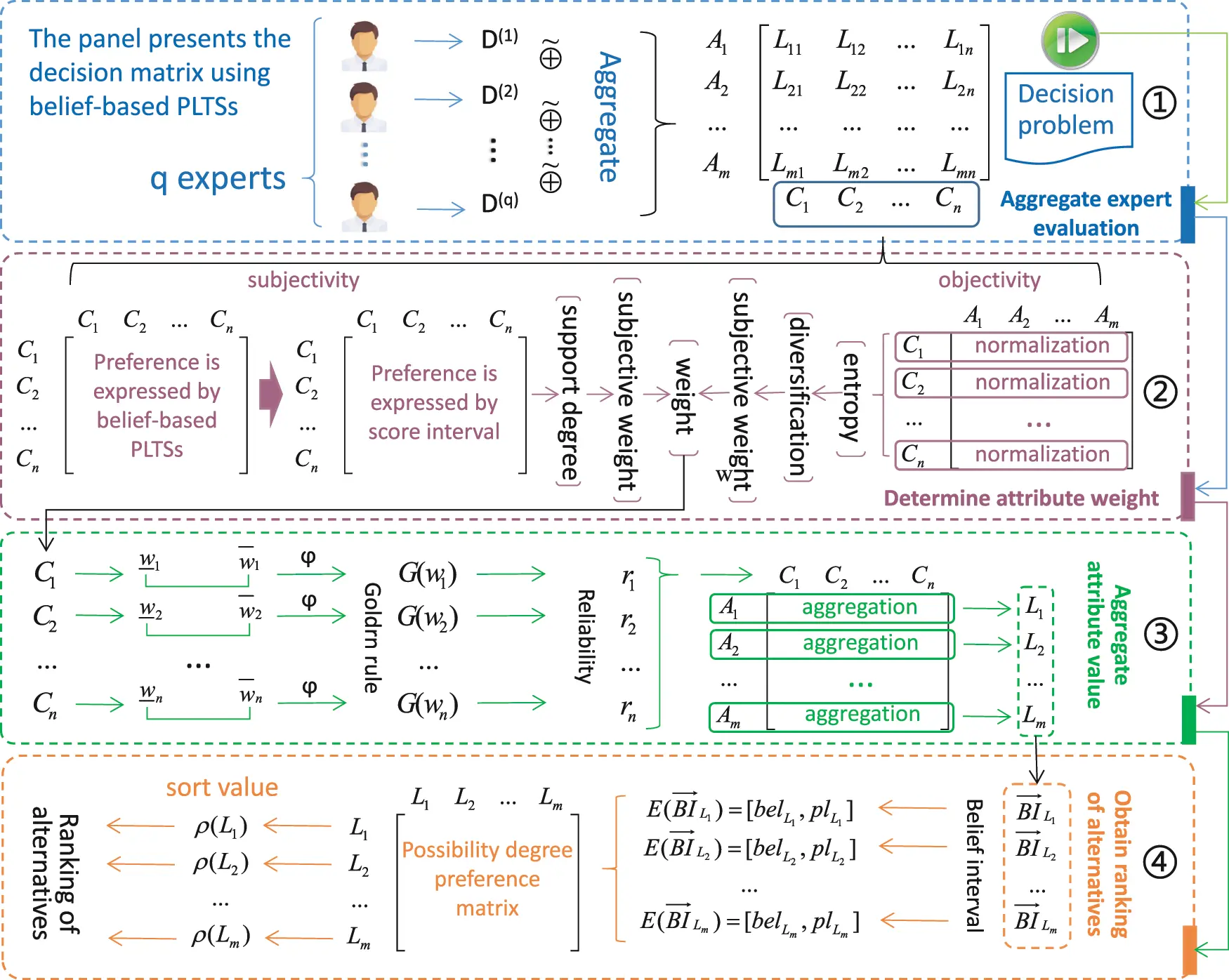Figure 2: Emergency decision-making framework based on belief-based PLTSs

5  Case Study

The emergency management bureau of municipality S (EMB-SM) is the agency responsible for the local government’s emergency management strategies. It coordinates and guides all districts in the city in responding to emergencies such as production safety, natural disasters, and comprehensive disaster prevention, mitigation and relief efforts. Citizen emergency education is an important step toward modernizing the emergency management system of municipality S. To this end, EMB-SM formulates three plans, namely, carrying out field exercises, developing emergency education apps, and disseminating emergency knowledge through propaganda manuals. In order to choose the most suitable of these three options, this section will demonstrate the effect of our proposed method based on a case study.

5.1 Decision Procedure

Based on the decision-making method in Section 4, the decision process of this case is shown as follows.

5.1.1 Problem Description

Three options (denoted as {A1=Drill,A2=APP(DigitalPlatform),A3=Brochures}) have been identified to educate citizens about disaster reduction, and the most suitable one needs to be selected. To select the best solution, three experts from EMB-SM (denoted as {E1,E2,E3}) evaluate them based on four attributes [4,63] (denoted as {C1 = ‘influence’, C2 = ‘coverage’, C3 = ‘interestingness’, C4 = ‘efficiency’}), and the results are recorded in the decision matrix, as shown in Table 3.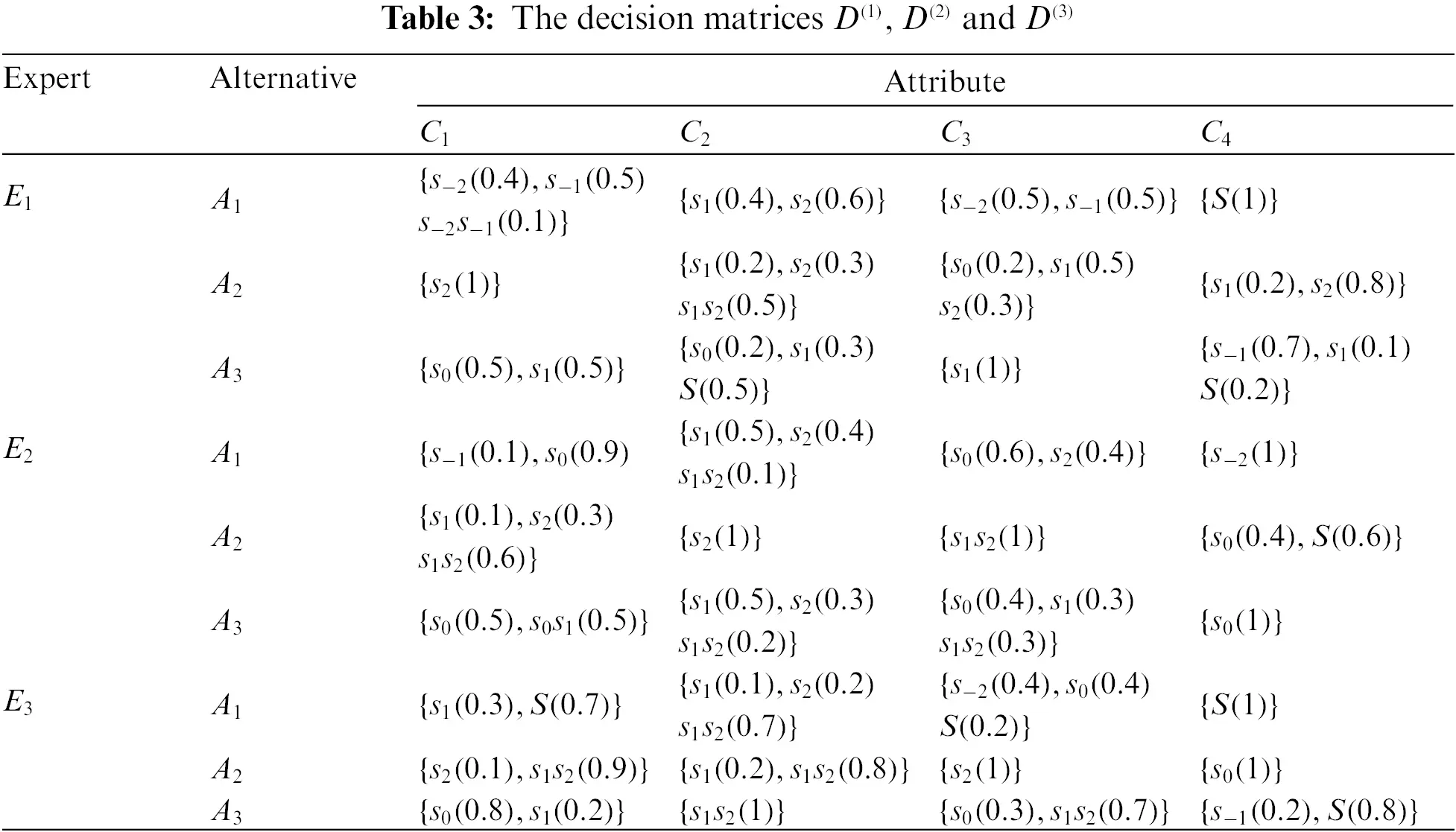To measure the level of suitability of emergency education plans, let the LTS be {s2: Not at all, s1: Not suitable for, s0: General, s1: Suitable for, s2: Extremely suitable for}, and belief-based PLTSs are used to express the evaluations in this study.

5.1.2 Aggregation of Decision Matrices from Different Experts

The decision matrices of D(1), D(2) and D(3) shown in Table 3 will be aggregated by using Definition 3.7, and in this case it is assumed that the experts have equal weight and α=0.5. The aggregated results are manifested in Table 4, which is the basis for subsequent decisions.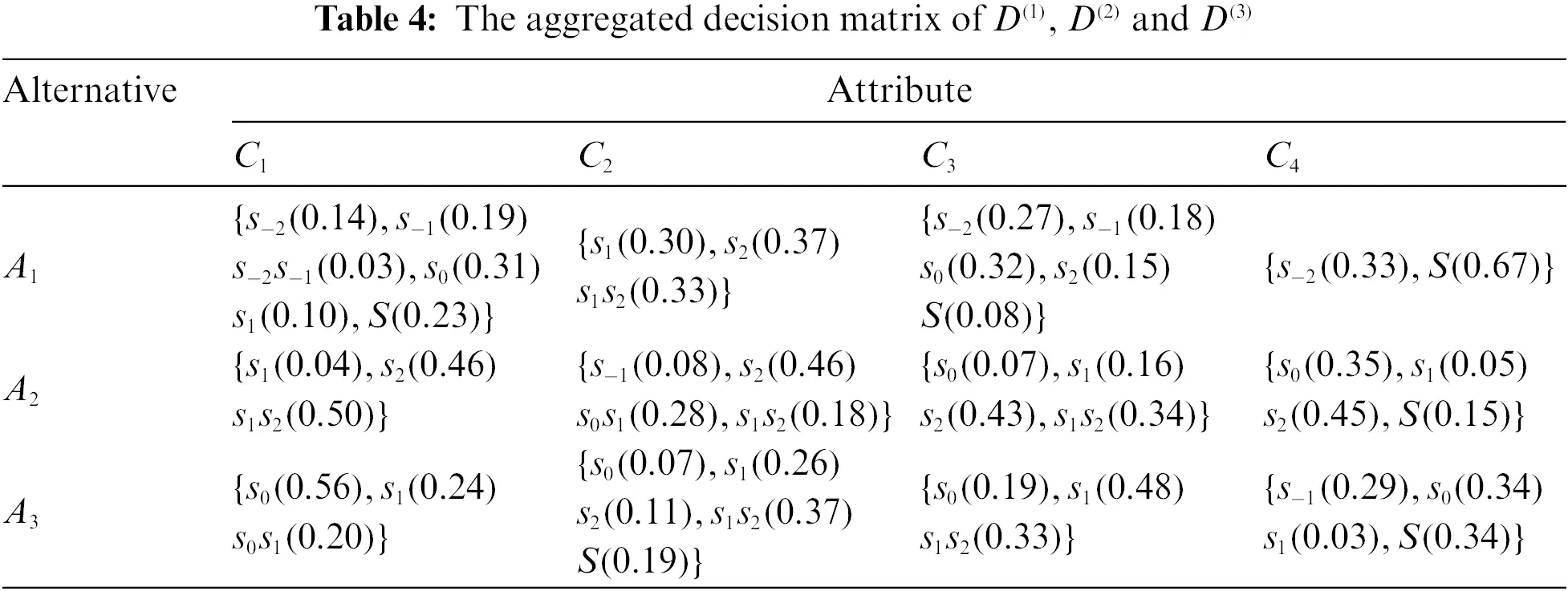5.1.3 Determination of Attribute Weight

According to Section 4.3, the determination of attribute weight is divided into two parts: subjective and objective. We first determine the subjective weight of the attribute. The pair comparison between attributes Ci and Cj (i<j) is provided by DMs, expressed as belief-based PLTSs. Based on Eq. (41), it is easy to calculate the negation of Ci and Cj, as shown in blue font in Table 5.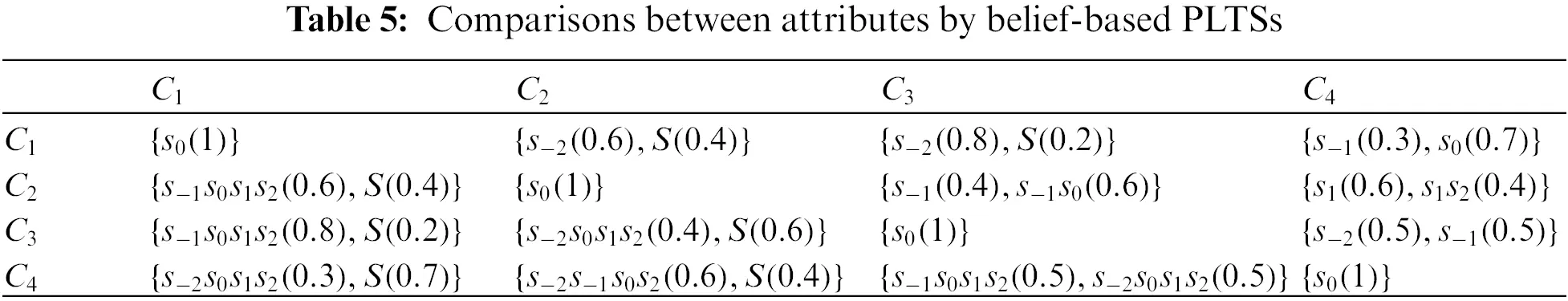Based on Definition 3.13, the score of ij can be calculated as [bel~ij𝒞,pl~ij𝒞], which are shown in Table 6. The support degree of an attribute can be determined as [sup1bel~,sup1pl~]=[3.7,4.9], [sup2bel~,sup2pl~]=[6.8,12.4], [sup3bel~,sup3pl~]=[5.5,9.5], and [sup4bel~,sup4pl~]=[4.8,11.6]. Then the subjective weight of attribute can be obtained as [w_1s,w¯1s]=[0.0964,0.1276], [w_2s,w¯2s]=[0.1771,0.3229], [w_3s,w¯3s]=[0.1432,0.2474], and [w_4s,w¯4s]=[0.1250,0.3021].In what follows, the objective weight will be determined based on the interval entropy weight method. Based on the aggregated decision matrix in Table 4, the score of the evaluation of alternative Ai under Cj can be calculated by employing Definition 3.13 and the results are shown in Table 7. The entropy of each attribute can be calculated based on Eq. (44) as [SE1belˇ,SE1belˇ]=[0.9151,0.9635], [SE2belˇ,SE2belˇ]=[0.9968,0.9987], [SE3belˇ,SE3belˇ]=[0.9483,0.9541], and [SE4belˇ,SE4belˇ]=[0.6003,0.9260]. Next, the diversification of each attribute can be calculated based on Eq. (45) as [div1belˇ,div1plˇ]=[0.0365,0.0849], [div2belˇ,div2plˇ]=[0.0013,0.0032], [div3belˇ,div3plˇ]=[0.0459,0.0517], and [div4belˇ,div4plˇ]=[0.0740,0.3997]. Therefore, the objective weight of each attribute can be calculated by using Eq. (46) as [w_1o,w¯1o]=[0.0677,0.1574], [w_2o,w¯2o]=[0.0024,0.0059], [w_3o,w¯3o]=[0.0851,0.0958], and [w_4o,w¯4o]=[0.1372,0.7409]. Finally, the comprehensive weight of each attribute can be calculated based on Eq. (47) by combining subjectivity and objectivity as w1=[w_1,w¯1]=[0.0242,0.0745], w2=[w_2,w¯2]=[0.0016,0.0071], w3=[w_3,w¯3]=[0.0452,0.0879], and w4=[w_4,w¯4]=[0.0452,0.8305].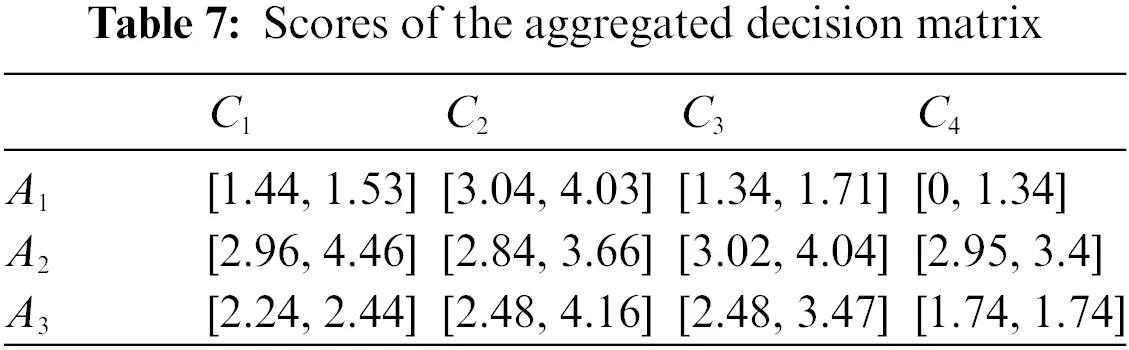5.1.4 Aggregation of Attribute Information

Based on the attribute weights obtained and the decision matrix in Table 4, the evaluations of each alternative under different attributes can be aggregated by the aggregation operator in Section 3.2. First, the golden rule is used to transform the interval weight, without loss of generality, let φ=0, the normalized weight is w1=0.0883, w2=0.0079, w3=0.1192, and w4=0.7846. The weight obtained can be considered as reliability, and the aggregated result of each alternative can be further calculated as 1={s2(0.2935),s1(0.0248),s0(0.0490),s1(0.0245),s2(0.0427),s2s1(0.0022),s1s2(0.0022),S(0.5611)}, 2={s1(0.0005),s0(0.2241),s1(0.1005),s2(0.5193),s0s1(0.0017),s1s2(0.0650),S(0.0889)}, 3={s1(0.1550),s0(0.4386),s1(0.1366),s2(0.0006),s0s1(0.0120),s1s2(0.0406),S(0.2166)}.

5.1.5 Calculation of the Ranking of Alternatives

According to the aggregated results, the final ranking of alternatives can be calculated by comparing the corresponding belief-based PLTSs. The sort value of Ai can be calculated based on Definition 4.3.1 as ρ(1)=0.2266, ρ(2)=0.4941, ρ(3)=0.2793. Therefore, the alternatives are sorted as A21A30.6754A1. Based on the method proposed in this study, it can be concluded that option A2 is the most suitable for emergency education, followed by A3 and A1. It was clear that option A2 has the highest priority, but the relationship between A3 and A1 is less obvious. Note that APP is indeed selected as the platform in the actual emergency education in S City, which is consistent with the results obtained in this paper and can demonstrate the effectiveness of the proposed method.

5.2 Sensitivity Analysis

The decision method proposed in this study involves several parameters, α in OWA operator and φ in the golden rule, respectively. In this section, the influence of parameters on the decision will be analyzed.

The parameter in the golden rule will be analyzed first. The function of the golden rule is to convert the interval weights into single values, where the parameter φ reflects the attitude of DMs. As can be seen from Fig. 3a, as the attitude of DMs becomes optimistic, more weight is allocated to w1, while the weights of other attributes decrease.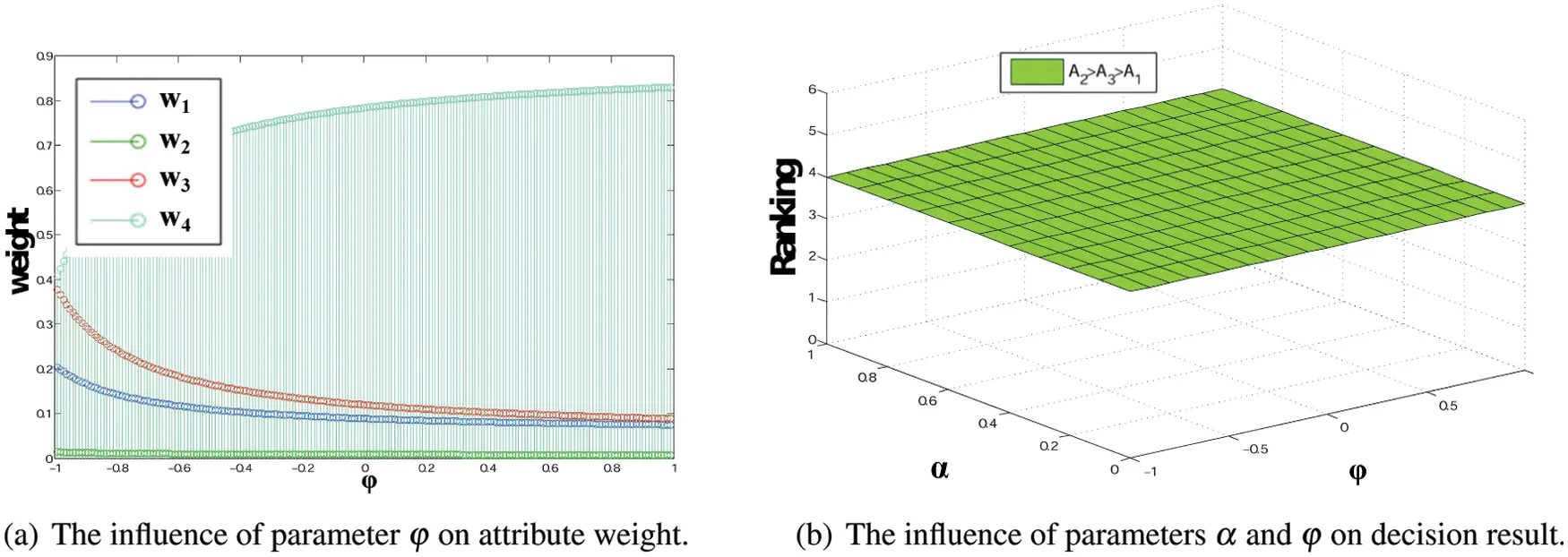Figure 3: Sensitivity analysis of decision results to parameters φ and α

In addition, we analyze the influence of parameters φ and α on the decision result. In this case, we need to prioritize 3 options, and there are 6 possible results, respectively (1) A1A2A3, (2) A1A3A2, (3) A2A1A3, (4) A2A3A1, (5) A3A1A2 and (6) A3A2A1. In Fig. 3b, we present the sorting result under the joint action of φ and α. It can be found that under different parameters, there is only one sorting result, namely A2A3A1, indicating that in this case, the sorting result is insensitive to parameters.

5.3 Comparison and Discussion

To further verify the effectiveness of the decision making method proposed in this paper, we conduct a group of comparative experiments and analyze the experimental results. We choose several decision methods with PLTS as the information expression to apply in the case in this paper. Since the data type in this study is belief-based PLTS, to adapt it to PLTS environment, we propose the following conversion method.

Definition 5.1. Let be a belief-based PLTS on S={sτ,,s1,s0,s1,,sτ}, it can be converted to a PLTS as

p(ι~)=ι2S,ι~ι|ι~ι||ι|m(ι),(50)

where |ι~| denotes the cardinality of  ι~.

Based on Definition 5.1, the belief-based PLTSs in Table 3 can be converted to PLTSs, as shown in Table 8. We will compare and analyze the different methods from the following aspects: aggregation operator, score function, attribute weight, applications, and ranking. The results of the comparison are shown in Table 9.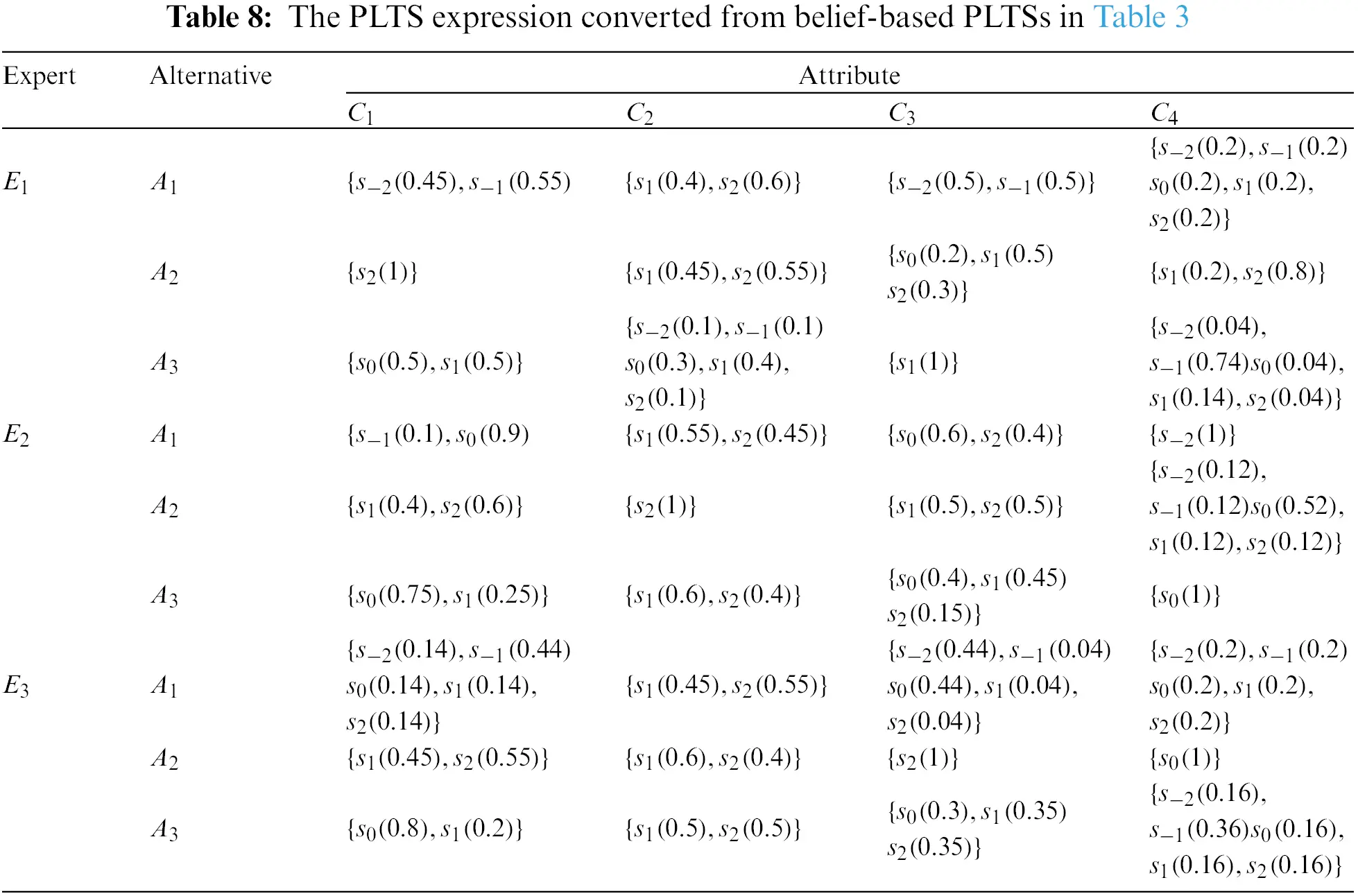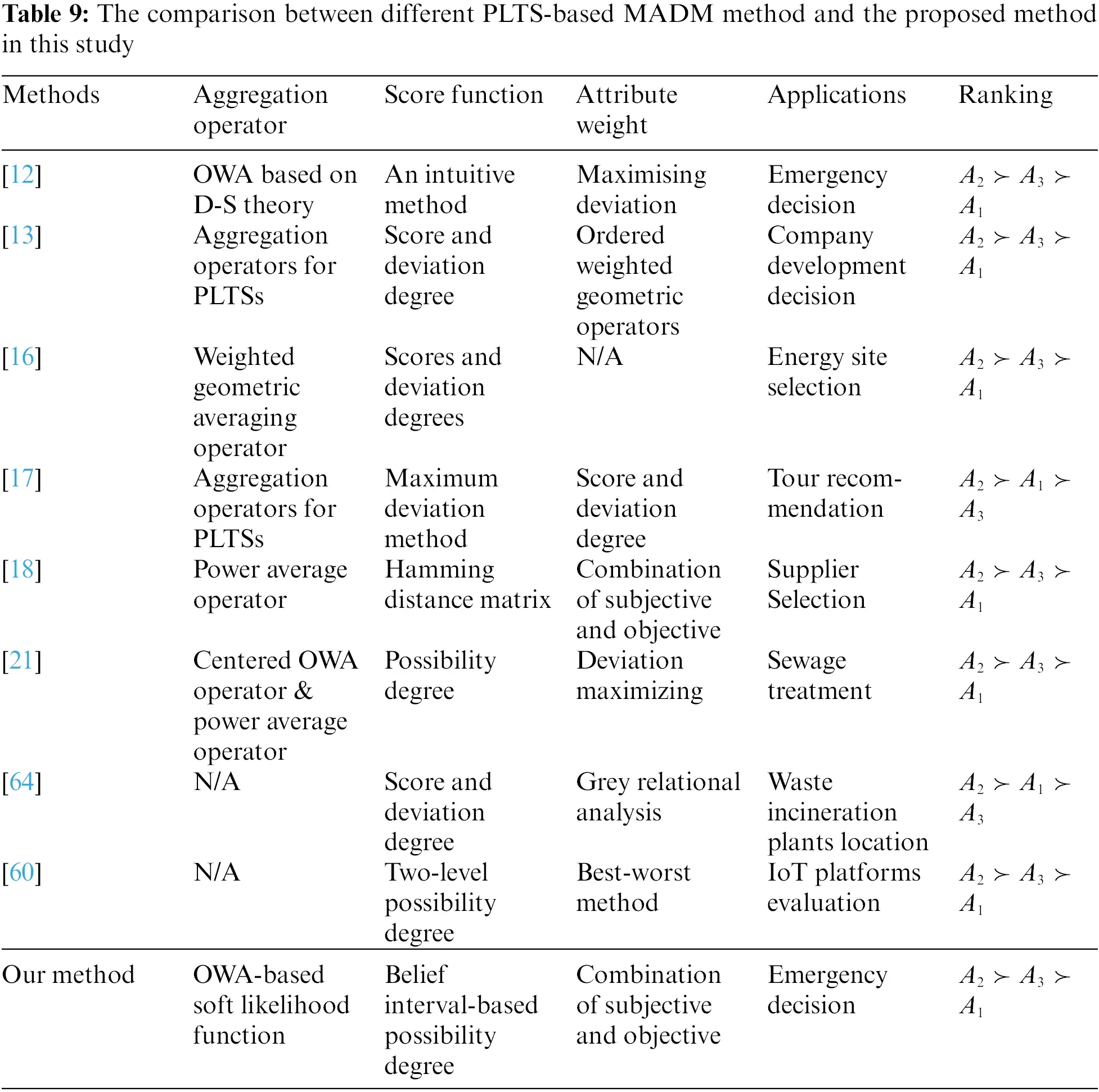Through comparison, it can be seen that the advantages of the proposed method in this paper are as follows. First, the scope of expression of probabilistic linguistic information is extended, and the related operations that can be used in decision-making, including the aggregation operator and score functions, are proposed. Further, a decision-making framework is developed. In addition, in the determination of attribute weights, we take both subjective and objective aspects into consideration to avoid deviations caused by subjective weighting and make use of all decision information. Although other methods have their own unique characteristics, the superiority of the method presented in this study is quite obvious. From the ranking of the results, all methods suggest that option A2 should be given the highest priority. Except for methods  and , A1 is superior to A3; all other methods rank A1 last. The above findings also verify the usefulness of our approach. In conclusion, this study offers many unique advantages in terms of its methods and results.

6  Conclusions and Future Directions

Through a careful analysis of the relevant literature, we found that the traditional PLTS method has deficiencies in its expression of uncertain information. To overcome this limitation, we incorporated the belief function theory into PLTS to construct belief-based PLTS and proposed a corresponding decision analysis approach that is able to address the aforementioned deficiencies through methods such as probability allocation on multiple linguistic terms. We found that the OWA-based soft likelihood function can be employed to define the aggregation operator of belief-based PLTS, which has the advantage of being able to fully describe decision makers’ attitudes. In the actual decision-making environment, belief-based PLTSs are usually reliable, so we also put forward an aggregation operator that considers reliability. We found that the belief interval can express more complete linguistic information, so we defined a score function that uses the belief interval to compare different belief-based PLTSs. According to the results, a multi-attribute decision-making framework was constructed for linguistic environments that is suitable for emergency decisions and can potentially be applied to other areas. Finally, an example was given to demonstrate the usefulness and effectiveness of the proposed method.

In future research, we aim to further develop these theories and methods based on belief-based PLTS. First, we will study a variety of operations to further refine their mathematical forms. Second, more methods, such as the analytic network process and the best-worst method, will be used to determine attribute weights in the decision-making framework. Third, the decision problem presented by the heterogeneous attributes of the belief-based PLTS environment will be further studied. Finally, attribute interactivity should be taken into account in future research.

Funding Statement: The work is partially supported by National Social Science Foundation of China (Grant No. 17ZDA030).

Conflicts of Interest: The authors declare that they have no conflicts of interest to report regarding the present study.

## References

1. Xu, X., Wang, L., Chen, X., & Liu, B. (2019). Large group emergency decision-making method with linguistic risk appetites based on criteria mining. Knowledge-Based Systems, 182, 104849. [Google Scholar] [CrossRef]
2. Hao, Z., Xu, Z., Zhao, H., & Fujita, H. (2017). A dynamic weight determination approach based on the intuitionistic fuzzy Bayesian network and its application to emergency decision making. IEEE Transactions on Fuzzy Systems, 26(4), 1893-1907. [Google Scholar] [CrossRef]
3. Karanci, A. N., Aksit, B., & Dirik, G. (2005). Impact of a community disaster awareness training program in Turkey: Does it influence hazard-related cognitions and preparedness behaviors. Social Behavior and Personality: An International Journal, 33(3), 243-258. [Google Scholar] [CrossRef]
4. Tsai, M. H., Chang, Y. L., Shiau, J. S., & Wang, S. M. (2020). Exploring the effects of a serious game-based learning package for disaster prevention education: The case of battle of flooding protection. International Journal of Disaster Risk Reduction, 43, 101393. [Google Scholar] [CrossRef]
5. Sun, B., Qi, C., Ma, W., Wang, T., & Zhang, L. (2020). Variable precision diversified attribute multigranulation fuzzy rough set-based multi-attribute group decision making problems. Computers & Industrial Engineering, 142, 106331. [Google Scholar] [CrossRef]
6. Xu, X., Zhang, Q., & Chen, X. (2020). Consensus-based non-cooperative behaviors management in large-group emergency decision-making considering experts’ trust relations and preference risks. Knowledge-Based Systems, 190, 105108. [Google Scholar] [CrossRef]
7. Zeng, S., Chen, S. M., & Fan, K. Y. (2020). Interval-valued intuitionistic fuzzy multiple attribute decision making based on nonlinear programming methodology and topsis method. Information Sciences, 506, 424-442. [Google Scholar] [CrossRef]
8. Ding, W., Wang, J., Wang, J. (2020). Multigranulation consensus fuzzy-rough based attribute reduction. Knowledge-Based Systems, 105945. DOI 10.1016/j.knosys.2020.105945. [CrossRef]
9. Zavadskas, E. K., Bausys, R., Lescauskiene, I., & Usovaite, A. (2021). Multimoora under interval-valued neutrosophic sets as the basis for the quantitative heuristic evaluation methodology hebin. Mathematics, 9(1), 66. [Google Scholar] [CrossRef]
10. Meng, F., Chen, S. M., & Tang, J. (2020). Group decision making based on acceptable multiplicative consistency of hesitant fuzzy preference relations. Information Sciences, 524, 77-96. [Google Scholar] [CrossRef]
11. Garg, H. (2020). Linguistic interval-valued pythagorean fuzzy sets and their application to multiple attribute group decision-making process. Cognitive Computation, 12(6), 1313-1337. [Google Scholar] [CrossRef]
12. Li, P., & Wei, C. (2019). An emergency decision-making method based on ds evidence theory for probabilistic linguistic term sets. International Journal of Disaster Risk Reduction, 37, 101178. [Google Scholar] [CrossRef]
13. Pang, Q., Wang, H., & Xu, Z. (2016). Probabilistic linguistic term sets in multi-attribute group decision making. Information Sciences, 369, 128-143. [Google Scholar] [CrossRef]
14. Liao, H., & Wu, X. (2020). DNMA: A double normalization-based multiple aggregation method for multi-expert multi-criteria decision making. Omega, 94, 102058. [Google Scholar] [CrossRef]
15. Jiang, L., & Liao, H. (2020). Mixed fuzzy least absolute regression analysis with quantitative and probabilistic linguistic information. Fuzzy Sets and Systems, 387, 35-48. [Google Scholar] [CrossRef]
16. Nie, R. X., & Wang, J. Q. (2020). Prospect theory-based consistency recovery strategies with multiplicative probabilistic linguistic preference relations in managing group decision making. Arabian Journal for Science and Engineering, 45(3), 2113-2130. [Google Scholar] [CrossRef]
17. Wang, X., Wang, J., & Zhang, H. (2019). Distance-based multicriteria group decision-making approach with probabilistic linguistic term sets. Expert Systems, 36(2), e12352. [Google Scholar] [CrossRef]
18. Wei, G., Wei, C., Wu, J., & Wang, H. (2019). Supplier selection of medical consumption products with a probabilistic linguistic mabac method. International Journal of Environmental Research and Public Health, 16(24), 5082. [Google Scholar] [CrossRef]
19. Li, B., Zhang, Y., & Xu, Z. (2020). The medical treatment service matching based on the probabilistic linguistic term sets with unknown attribute weights. International Journal of Fuzzy Systems, 22, 1487-1505. [Google Scholar] [CrossRef]
20. Liang, D., Dai, Z., Wang, M., & Li, J. (2020). Web celebrity shop assessment and improvement based on online review with probabilistic linguistic term sets by using sentiment analysis and fuzzy cognitive map. Fuzzy Optimization and Decision Making, 19, 561-586. [Google Scholar] [CrossRef]
21. Feng, X., Zhang, Q., & Jin, L. (2019). Aggregation of pragmatic operators to support probabilistic linguistic multi-criteria group decision-making problems. Soft Computing, 24, 7735-7755. [Google Scholar]
22. Xie, W., Xu, Z., Ren, Z., Herrera-Viedma, E. (2020). A new multi-criteria decision model based on incomplete dual probabilistic linguistic preference relations. Applied Soft Computing, 106237. DOI 10.1016/j.asoc.2020.106237. [CrossRef]
23. Yang, J. B., Wang, Y. M., Xu, D. L., & Chin, K. S. (2006). The evidential reasoning approach for mada under both probabilistic and fuzzy uncertainties. European Journal of Operational Research, 171(1), 309-343. [Google Scholar] [CrossRef]
24. Fang, R., Liao, H., Yang, J. B., & Xu, D. L. (2021). Generalised probabilistic linguistic evidential reasoning approach for multi-criteria decision-making under uncertainty. Journal of the Operational Research Society, 72(1), 130-144. [Google Scholar] [CrossRef]
25. Lin, M., Xu, Z., Zhai, Y., & Yao, Z. (2017). Multi-attribute group decision-making under probabilistic uncertain linguistic environment. Journal of the Operational Research Society, 68, 1-15. [Google Scholar]
26. Mi, X., Liao, H., Wu, X., & Xu, Z. (2020). Probabilistic linguistic information fusion: A survey on aggregation operators in terms of principles, definitions, classifications, applications, and challenges. International Journal of Intelligent Systems, 35(3), 529-556. [Google Scholar] [CrossRef]
27. Liao, H., Mi, X., & Xu, Z. (2020). A survey of decision-making methods with probabilistic linguistic information: Bibliometrics, preliminaries, methodologies, applications and future directions. Fuzzy Optimization and Decision Making, 19(1), 81-134. [Google Scholar] [CrossRef]
28. Fei, L., & Feng, Y. (2021). Modeling interactive multiattribute decision-making via probabilistic linguistic term set extended by Dempster–Shafer theory. International Journal of Fuzzy Systems, 23(2), 599-613. [Google Scholar] [CrossRef]
29. Dempster, A. P. (1967). Upper and lower probabilities induced by a multivalued mapping. Annals of Mathematics and Statistics, 38(2), 325-339. [Google Scholar] [CrossRef]
30. Shafer, G. (1976). A mathematical theory of evidence. Princeton: Princeton University Press.
31. Wu, X., Liao, H., Zavadskas, E. K., & Antuchevičienė, J. (2022). A probabilistic linguistic vikor method to solve mcdm problems with inconsistent criteria for different alternatives. Technological and Economic Development of Economy, 28(2), 559-580. [Google Scholar] [CrossRef]
32. Deng, Y. (2020). Information volume of mass function. International Journal of Computers, Communications & Control, 15(6), [Google Scholar] [CrossRef]
33. Xiao, F. (2021). CEQD: A complex mass function to predict interference effects. IEEE Transactions on Cybernetics, 52(8), 7402-7414. [Google Scholar]
34. Deng, Y. (2020). Uncertainty measure in evidence theory. Science China Information Sciences, 63(11), 1-19. [Google Scholar] [CrossRef]
35. Xiao, F. (2021). CaFtR: A fuzzy complex event processing method. International Journal of Fuzzy Systems, 1–14. DOI 10.1007/s40815–021–01118–6. [CrossRef]
36. Deng, X., & Jiang, W. (2020). On the negation of a Dempster–Shafer belief structure based on maximum uncertainty allocation. Information Sciences, 516, 346-352. [Google Scholar] [CrossRef]
37. Zhang, H., Jiang, W., & Deng, X. (2020). Data-driven multi-attribute decision-making by combining probability distributions based on compatibility and entropy. Applied Intelligence, 50(11), 4081-4093. [Google Scholar] [CrossRef]
38. Mahmood, T., & Ali, Z. (2020). Aggregation operators and vikor method based on complex q-rung orthopair uncertain linguistic informations and their applications in multi-attribute decision making. Computational and Applied Mathematics, 39(4), 1-44. [Google Scholar] [CrossRef]
39. Xu, Z. (2005). Deviation measures of linguistic preference relations in group decision making. Omega, 33(3), 249-254. [Google Scholar] [CrossRef]
40. Deng, Y. (2022). Random permutation set. International Journal of Computers Communications & Control, 17(1), 4542. [Google Scholar] [CrossRef]
41. Ding, W., Lin, C. T., Liew, A. W. C., Triguero, I., & Luo, W. (2020). Current trends of granular data mining for biomedical data analysis. Information Sciences, 510, 341-343. [Google Scholar] [CrossRef]
42. Fang, R., Liao, H., & Mardani, A. (2022). How to aggregate uncertain and incomplete cognitive evaluation information in lung cancer treatment plan selection? A method based on dempster-shafer theory. Information Sciences, 603, 222-243. [Google Scholar] [CrossRef]
43. Qiang, C., Deng, Y., & Cheong, K. H. (2022). Information fractal dimension of mass function. Fractals, 30(6), 2250110. [Google Scholar] [CrossRef]
44. Zhou, Q., Deng, Y., & Pedrycz, W. (2022). Information dimension of Galton board. Fractals, 30(4), 2250079. [Google Scholar] [CrossRef]
45. Liu, R., Fei, L., & Mi, J. (2022). An evidential multimoora approach to assessing disaster risk reduction education strategies under a heterogeneous linguistic environment. International Journal of Disaster Risk Reduction, 78, 103114. [Google Scholar] [CrossRef]
46. Denoeux, T., & Shenoy, P. P. (2020). An interval-valued utility theory for decision making with dempster-shafer belief functions. International Journal of Approximate Reasoning, 124, 194-216. [Google Scholar] [CrossRef]
47. Deng, Z., & Wang, J. (2020). A novel decision probability transformation method based on belief interval. Knowledge-Based Systems, 208, 106427. [Google Scholar] [CrossRef]
48. Yager, R. R. (1988). On ordered weighted averaging aggregation operators in multicriteria decisionmaking. IEEE Transactions on Systems, Man, and Cybernetics, 18(1), 183-190. [Google Scholar] [CrossRef]
49. Jin, L., Mesiar, R., Kalina, M., & Yager, R. R. (2020). Canonical form of ordered weighted averaging operators. Annals of Operations Research, 295(2), 605-631. [Google Scholar] [CrossRef]
50. Jin, L., Mesiar, R., & Yager, R. (2020). Parameterized preference aggregation operators with improved adjustability. International Journal of General Systems, 49(8), 843-855. [Google Scholar] [CrossRef]
51. Yager, R. R. (1996). Quantifier guided aggregation using owa operators. International Journal of Intelligent Systems, 11(1), 49-73. [Google Scholar] [CrossRef]
52. Klir, G. J., Wierman, M. J. (2013). Uncertainty-based information: Elements of generalized information theory, vol. 15. New York: Physica.
53. Yager, R. R., Elmore, P., & Petry, F. (2017). Soft likelihood functions in combining evidence. Information Fusion, 36, 185-190. [Google Scholar] [CrossRef]
54. Zhu, R., Liu, Q., Huang, C., & Kang, B. (2022). Z-ACM: An approximate calculation method of z-numbers for large data sets based on kernel density estimation and its application in decision-making. Information Sciences, 610, 440-471. [Google Scholar] [CrossRef]
55. Flores-Sosa, M., Avilés-Ochoa, E., Merigó, J. M., & Yager, R. R. (2021). Volatility garch models with the ordered weighted average (OWA) operators. Information Sciences, 565, 46-61. [Google Scholar] [CrossRef]
56. Mi, X., Tian, Y., & Kang, B. (2020). A modified soft-likelihood function based on powa operator. International Journal of Intelligent Systems, 35(5), 869-890. [Google Scholar] [CrossRef]
57. Fei, L., Wang, Y. (2022). An optimization model for rescuer assignments under an uncertain environment by using dempster-shafer theory. Knowledge-Based Systems, 109680. DOI 10.1016/j.knosys.2022.109680. [CrossRef]
58. Fei, L., & Feng, Y. (2021). A dynamic framework of multi-attribute decision making under pythagorean fuzzy environment by using Dempster–Shafer theory. Engineering Applications of Artificial Intelligence, 101, 104213. [Google Scholar] [CrossRef]
59. Xu, Z., & Da, Q. (2003). Possibility degree method for ranking interval numbers and its application. Journal of Systems Engineering, 18(1), 67-70. [Google Scholar]
60. Lin, M., Huang, C., Xu, Z., & Chen, R. (2020). Evaluating iot platforms using integrated probabilistic linguistic mcdm method. IEEE Internet of Things Journal, 7(11), 11195-11208. [Google Scholar] [CrossRef]
61. Yager, R. R. (2014). On the maximum entropy negation of a probability distribution. IEEE Transactions on Fuzzy Systems, 23(5), 1899-1902. [Google Scholar] [CrossRef]
62. Fei, L. (2019). On interval-valued fuzzy decision-making using soft likelihood functions. International Journal of Intelligent Systems, 34(7), 1631-1652. [Google Scholar] [CrossRef]
63. Kraiger, K., Ford, J. K., & Salas, E. (1993). Application of cognitive, skill-based, and affective theories of learning outcomes to new methods of training evaluation. Journal of Applied Psychology, 78(2), 311-328. [Google Scholar] [CrossRef]
64. Lei, F., Wei, G., Lu, J., Wu, J., & Wei, C. (2019). Gra method for probabilistic linguistic multiple attribute group decision making with incomplete weight information and its application to waste incineration plants location problem. International Journal of Computational Intelligence Systems, 12(2), 1547-1556. [Google Scholar] [CrossRef]

Liu, R., Fei, L., Mi, J. (2023). A Multi-Attribute Decision-Making Method Using Belief-Based Probabilistic Linguistic Term Sets and Its Application in Emergency Decision-Making. CMES-Computer Modeling in Engineering & Sciences, 136(2), 2039–2067.This work is licensed under a Creative Commons Attribution 4.0 International License , which permits unrestricted use, distribution, and reproduction in any medium, provided the original work is properly cited.

View

Like

## Related articles

• Chak Fong Cheang, Yiqin Wang,...
• Haoran Huang, Qinyong Lin, Weitong...
• Shouzhen Zeng, Zeeshan Ali, Tahir...
• Changxing Fan, Jihong Chen, Keli...
• Xiaobing Mao, Hao Wu, Shuping...